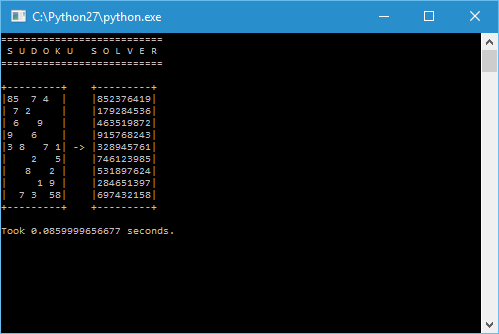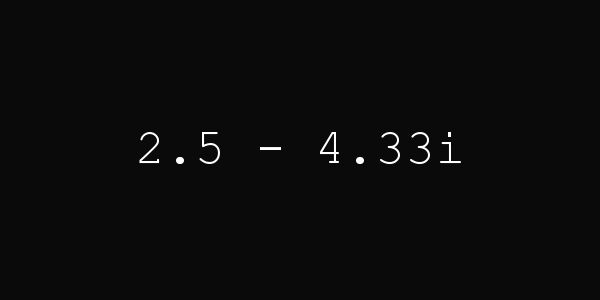# Web Sudoku Solver

2016-08-13, post № 137

Auto Hotkey, games, programming, Python, #AHK, #automatic, #fast times, #good times, #web, #Web Sudoku

In my last post I used Web Sudoku to get a Sudoku as an example for my solver.

After that I wanted to automate the process of looking up a Sudoku, solving it and typing it in. But while trying to get the Sudoku’s numbers, I noticed that the whole, solved Sudoku was stored in plaintext! (Look at this page’s source code.)
So I just needed to get that information, open the Web Sudoku page in a browser and type in the already solved Sudoku.

To accomplish said goal I used the python module urllib to get the Web Sudoku page’s source code and the module webbrowser to open the page in a browser. To type in the Sudoku I used AutoHotkey.

The finished program takes a level (easy, medium, hard or evil) and an id (the Sudoku’s identification number) to get a Sudoku, create an AHK file, execute it and open a web browser.
All you have to do is to click into the first box, press a key (‘F1’ in this case) and the Sudoku gets solved! You then just need to wait a minute, which is the minimum time Web Sudoku wants you to take to solve a Sudoku, and the AHK script hits enter.

You can get really good times with this:

Source code: web-sudoku-solver.py

# Sudoku Solver

2016-08-06, post № 136

programming, Python, #666, #evil, #logic, #solve, #solving, #Web Sudoku

This program solves a given Sudoku.
It uses a simple strategy, looking at each box and determining those numbers that are not in its row, column and square. If that list has length 𝟣, the box’s number is determined.
After going through each box, the program generates child Sudokus in which the first empty box get filled with one of the possible numbers for that box. Sudokus with an error get eliminated.
Using this simple but effective strategy this program can solve hard Sudokus in under a second. As an example, I used Evil Puzzle 666 from Web Sudoku.
For more information on Sudokus, visit this Wikipedia entry.Source code: sudoku-solver.py

# JClock VIII

2016-07-30, post № 135

programming, Pygame, Python, #clock, #complex number, #hour, #minute, #plane, #time

Interpreting the hour hand on a clock as a two-dimensional object on a plane, the hand’s tip can be seen as a complex number.
This clock converts the hour hand’s position into a complex number, sets the number’s length to the current minutes and displays it in the form$a+b\cdot i$.
The angle 𝜑 is determined by the hours passed ($\frac{2\cdot\pi\cdot\text{hour}}{12}=\frac{\pi\cdot\text{hour}}{6}$) but has to be slightly modified because a complex number starts at the horizontal axis and turns anti-clockwise whilst an hour hand starts at the vertical axis and turns — as the name implies — clockwise. Thus,$\varphi=(2\cdot\pi-\frac{\pi\cdot\text{hour}}{6})+\frac{\pi}{2}=(\frac{15-\text{hour}}{6})\cdot\pi$.

The complex number’s length is simply determined by the minutes passed. Because the length must not be equal to 𝟢, I simply add 𝟣:$|z|=k=\text{minute}+1$.
Lastly, to convert a complex number of the form$k\cdot e^{\varphi\cdot i}$ into the form$a+b\cdot i$, I use the formula$k\cdot(\cos{\varphi}+\sin{\varphi}\cdot i)=a+b\cdot i$.Source code: jclock-viii.py
Jonathan Frech's blog; built 2021/04/16 20:21:20 CEST